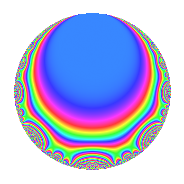Properties

 Label 8016.2.a.oLevel 8016 Weight 2 Character orbit 8016.a Self dual Yes Analytic conductor 64.008 Analytic rank 0 Dimension 4 CM No Inner twists 1

Related objects

Newspace parameters

 Level: $$N$$ = $$8016 = 2^{4} \cdot 3 \cdot 167$$ Weight: $$k$$ = $$2$$ Character orbit: $$[\chi]$$ = 8016.a (trivial)

Newform invariants

 Self dual: Yes Analytic conductor: $$64.0080822603$$ Analytic rank: $$0$$ Dimension: $$4$$ Coefficient field: 4.4.2777.1 Coefficient ring: $$\Z[a_1, \ldots, a_{13}]$$ Coefficient ring index: $$2^{2}$$ Fricke sign: $$-1$$ Sato-Tate group: $\mathrm{SU}(2)$

$q$-expansion

Coefficients of the $$q$$-expansion are expressed in terms of a basis $$1,\beta_1,\beta_2,\beta_3$$ for the coefficient ring described below. We also show the integral $$q$$-expansion of the trace form.

 $$f(q)$$ $$=$$ $$q$$ $$+ q^{3}$$ $$+ ( 1 - \beta_{1} ) q^{5}$$ $$+ ( -\beta_{2} + \beta_{3} ) q^{7}$$ $$+ q^{9}$$ $$+O(q^{10})$$ $$q$$ $$+ q^{3}$$ $$+ ( 1 - \beta_{1} ) q^{5}$$ $$+ ( -\beta_{2} + \beta_{3} ) q^{7}$$ $$+ q^{9}$$ $$+ ( 2 + \beta_{3} ) q^{13}$$ $$+ ( 1 - \beta_{1} ) q^{15}$$ $$+ ( 3 + \beta_{1} - \beta_{2} ) q^{17}$$ $$+ 2 \beta_{2} q^{19}$$ $$+ ( -\beta_{2} + \beta_{3} ) q^{21}$$ $$-2 \beta_{2} q^{23}$$ $$+ ( 1 - 2 \beta_{1} - \beta_{2} + \beta_{3} ) q^{25}$$ $$+ q^{27}$$ $$+ ( 4 + 2 \beta_{1} ) q^{29}$$ $$+ ( -\beta_{2} - \beta_{3} ) q^{31}$$ $$+ ( -2 - 2 \beta_{1} + \beta_{2} + \beta_{3} ) q^{35}$$ $$+ ( 2 - 3 \beta_{2} ) q^{37}$$ $$+ ( 2 + \beta_{3} ) q^{39}$$ $$+ ( 3 + \beta_{1} + 3 \beta_{2} ) q^{41}$$ $$+ ( -1 - \beta_{1} + \beta_{2} - \beta_{3} ) q^{43}$$ $$+ ( 1 - \beta_{1} ) q^{45}$$ $$+ ( -2 - 2 \beta_{1} - \beta_{2} + \beta_{3} ) q^{47}$$ $$+ ( 3 + 2 \beta_{1} + 3 \beta_{2} - \beta_{3} ) q^{49}$$ $$+ ( 3 + \beta_{1} - \beta_{2} ) q^{51}$$ $$+ ( 1 - \beta_{1} - 2 \beta_{2} ) q^{53}$$ $$+ 2 \beta_{2} q^{57}$$ $$+ ( 2 + 2 \beta_{1} - \beta_{2} + 2 \beta_{3} ) q^{59}$$ $$-2 \beta_{1} q^{61}$$ $$+ ( -\beta_{2} + \beta_{3} ) q^{63}$$ $$+ ( -4 \beta_{1} + 2 \beta_{2} + 2 \beta_{3} ) q^{65}$$ $$+ ( 3 + 3 \beta_{1} - 2 \beta_{2} - 2 \beta_{3} ) q^{67}$$ $$-2 \beta_{2} q^{69}$$ $$+ ( -2 - 2 \beta_{1} + 4 \beta_{2} ) q^{71}$$ $$+ ( -2 \beta_{1} - 2 \beta_{3} ) q^{73}$$ $$+ ( 1 - 2 \beta_{1} - \beta_{2} + \beta_{3} ) q^{75}$$ $$+ ( 3 + 3 \beta_{1} + \beta_{2} ) q^{79}$$ $$+ q^{81}$$ $$+ ( -4 - 4 \beta_{1} - \beta_{2} ) q^{83}$$ $$+ ( -2 - 2 \beta_{1} - 2 \beta_{3} ) q^{85}$$ $$+ ( 4 + 2 \beta_{1} ) q^{87}$$ $$+ ( 4 + 2 \beta_{1} - \beta_{2} - \beta_{3} ) q^{89}$$ $$+ ( 8 - 2 \beta_{2} + 2 \beta_{3} ) q^{91}$$ $$+ ( -\beta_{2} - \beta_{3} ) q^{93}$$ $$+ ( 2 \beta_{2} + 2 \beta_{3} ) q^{95}$$ $$+ ( 2 + 4 \beta_{1} + \beta_{2} - \beta_{3} ) q^{97}$$ $$+O(q^{100})$$ $$\operatorname{Tr}(f)(q)$$ $$=$$ $$4q$$ $$\mathstrut +\mathstrut 4q^{3}$$ $$\mathstrut +\mathstrut 5q^{5}$$ $$\mathstrut -\mathstrut q^{7}$$ $$\mathstrut +\mathstrut 4q^{9}$$ $$\mathstrut +\mathstrut O(q^{10})$$ $$4q$$ $$\mathstrut +\mathstrut 4q^{3}$$ $$\mathstrut +\mathstrut 5q^{5}$$ $$\mathstrut -\mathstrut q^{7}$$ $$\mathstrut +\mathstrut 4q^{9}$$ $$\mathstrut +\mathstrut 8q^{13}$$ $$\mathstrut +\mathstrut 5q^{15}$$ $$\mathstrut +\mathstrut 10q^{17}$$ $$\mathstrut +\mathstrut 2q^{19}$$ $$\mathstrut -\mathstrut q^{21}$$ $$\mathstrut -\mathstrut 2q^{23}$$ $$\mathstrut +\mathstrut 5q^{25}$$ $$\mathstrut +\mathstrut 4q^{27}$$ $$\mathstrut +\mathstrut 14q^{29}$$ $$\mathstrut -\mathstrut q^{31}$$ $$\mathstrut -\mathstrut 5q^{35}$$ $$\mathstrut +\mathstrut 5q^{37}$$ $$\mathstrut +\mathstrut 8q^{39}$$ $$\mathstrut +\mathstrut 14q^{41}$$ $$\mathstrut -\mathstrut 2q^{43}$$ $$\mathstrut +\mathstrut 5q^{45}$$ $$\mathstrut -\mathstrut 7q^{47}$$ $$\mathstrut +\mathstrut 13q^{49}$$ $$\mathstrut +\mathstrut 10q^{51}$$ $$\mathstrut +\mathstrut 3q^{53}$$ $$\mathstrut +\mathstrut 2q^{57}$$ $$\mathstrut +\mathstrut 5q^{59}$$ $$\mathstrut +\mathstrut 2q^{61}$$ $$\mathstrut -\mathstrut q^{63}$$ $$\mathstrut +\mathstrut 6q^{65}$$ $$\mathstrut +\mathstrut 7q^{67}$$ $$\mathstrut -\mathstrut 2q^{69}$$ $$\mathstrut -\mathstrut 2q^{71}$$ $$\mathstrut +\mathstrut 2q^{73}$$ $$\mathstrut +\mathstrut 5q^{75}$$ $$\mathstrut +\mathstrut 10q^{79}$$ $$\mathstrut +\mathstrut 4q^{81}$$ $$\mathstrut -\mathstrut 13q^{83}$$ $$\mathstrut -\mathstrut 6q^{85}$$ $$\mathstrut +\mathstrut 14q^{87}$$ $$\mathstrut +\mathstrut 13q^{89}$$ $$\mathstrut +\mathstrut 30q^{91}$$ $$\mathstrut -\mathstrut q^{93}$$ $$\mathstrut +\mathstrut 2q^{95}$$ $$\mathstrut +\mathstrut 5q^{97}$$ $$\mathstrut +\mathstrut O(q^{100})$$

Basis of coefficient ring in terms of a root $$\nu$$ of $$x^{4}\mathstrut -\mathstrut$$ $$x^{3}\mathstrut -\mathstrut$$ $$4$$ $$x^{2}\mathstrut +\mathstrut$$ $$x\mathstrut +\mathstrut$$ $$2$$:

 $$\beta_{0}$$ $$=$$ $$1$$ $$\beta_{1}$$ $$=$$ $$\nu^{3} - \nu^{2} - 2 \nu$$ $$\beta_{2}$$ $$=$$ $$\nu^{3} - \nu^{2} - 4 \nu + 1$$ $$\beta_{3}$$ $$=$$ $$2 \nu^{2} - 2 \nu - 4$$
 $$1$$ $$=$$ $$\beta_0$$ $$\nu$$ $$=$$ $$($$$$-$$$$\beta_{2}\mathstrut +\mathstrut$$ $$\beta_{1}\mathstrut +\mathstrut$$ $$1$$$$)/2$$ $$\nu^{2}$$ $$=$$ $$($$$$\beta_{3}\mathstrut -\mathstrut$$ $$\beta_{2}\mathstrut +\mathstrut$$ $$\beta_{1}\mathstrut +\mathstrut$$ $$5$$$$)/2$$ $$\nu^{3}$$ $$=$$ $$($$$$\beta_{3}\mathstrut -\mathstrut$$ $$3$$ $$\beta_{2}\mathstrut +\mathstrut$$ $$5$$ $$\beta_{1}\mathstrut +\mathstrut$$ $$7$$$$)/2$$

Embeddings

For each embedding $$\iota_m$$ of the coefficient field, the values $$\iota_m(a_n)$$ are shown below.

For more information on an embedded modular form you can click on its label.

Label $$\iota_m(\nu)$$ $$a_{2}$$ $$a_{3}$$ $$a_{4}$$ $$a_{5}$$ $$a_{6}$$ $$a_{7}$$ $$a_{8}$$ $$a_{9}$$ $$a_{10}$$
1.1
 2.36234 −0.679643 0.825785 −1.50848
0 1.00000 0 −1.87806 0 3.28324 0 1.00000 0
1.2 0 1.00000 0 0.416566 0 −4.65960 0 1.00000 0
1.3 0 1.00000 0 2.77037 0 −1.86579 0 1.00000 0
1.4 0 1.00000 0 3.69113 0 2.24216 0 1.00000 0
 $$n$$: e.g. 2-40 or 990-1000 Significant digits: Format: Complex embeddings Normalized embeddings Satake parameters Satake angles

Inner twists

This newform does not admit any (nontrivial) inner twists.

Atkin-Lehner signs

$$p$$ Sign
$$2$$ $$-1$$
$$3$$ $$-1$$
$$167$$ $$-1$$

Hecke kernels

This newform can be constructed as the intersection of the kernels of the following linear operators acting on $$S_{2}^{\mathrm{new}}(\Gamma_0(8016))$$:

 $$T_{5}^{4}$$ $$\mathstrut -\mathstrut 5 T_{5}^{3}$$ $$\mathstrut +\mathstrut 20 T_{5}$$ $$\mathstrut -\mathstrut 8$$ $$T_{7}^{4}$$ $$\mathstrut +\mathstrut T_{7}^{3}$$ $$\mathstrut -\mathstrut 20 T_{7}^{2}$$ $$\mathstrut +\mathstrut 64$$ $$T_{11}$$ $$T_{13}^{4}$$ $$\mathstrut -\mathstrut 8 T_{13}^{3}$$ $$\mathstrut +\mathstrut 4 T_{13}^{2}$$ $$\mathstrut +\mathstrut 56 T_{13}$$ $$\mathstrut -\mathstrut 16$$